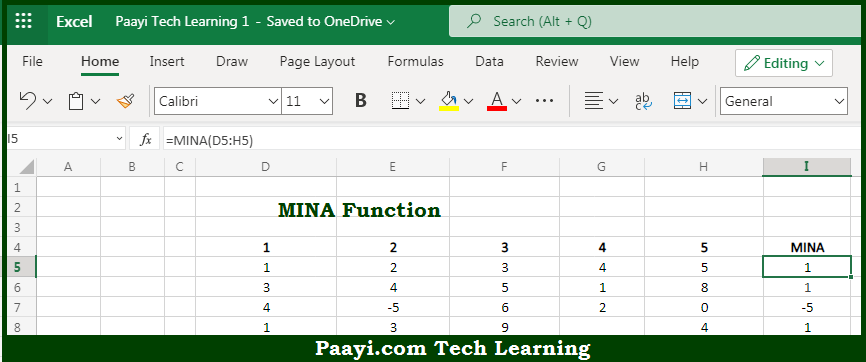# Learn How to Use Microsoft Excel MINA Function

Written by | 0 Comments | 562 Views

In this article, you will learn how to use the Microsoft Excel MINA function and its prime function in Microsoft Excel. You will also get to know the Microsoft Excel MINA function return value and syntax with the help of some examples.

Microsoft Excel MINA Function

The main purpose of the Microsoft Excel MINA function is to return the smallest value from the given array. That implies, with the help of the MINA function you can able to return the smallest numeric value in a range of values. The MINA function ignores empty cells, the logical values TRUE and FALSE, as 1 and 0 respectively. So, with the help of the MINA function, you can able to get the smallest value from the range of values provided.

Return Value of MINA Function

The return value will be the smallest numeric value.

Syntax of MINA Function

=MINA(value1, [value], ...)

Where the arguments:

• value1: This is the number, reference to a numeric value or range that contains numeric values.
• value2: This is the number, reference to a numeric value or range that contains numeric values (optional).

## How to Use Microsoft Excel MINA Function?So we know that Microsoft Excel MINA function you can able to return the smallest value from the given array. That implies, with the help of the MINA function you can able to return the smallest numeric value in a range of values. The MINA function ignores empty cells, the logical values TRUE and FALSE, as 1 and 0 respectively. So, with the help of the MINA function, you can able to get the smallest value from the range of values provided.